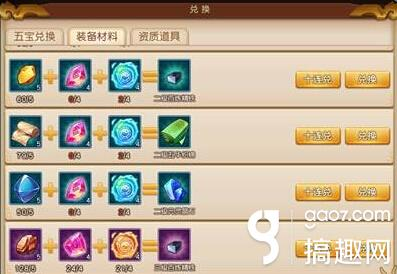梦幻西游无双2中当玩家达到60级之后肯定深深地感受到了60装备材料的稀缺，不过面包总会有的，慢慢积累总会有,那么下面就跟小编一起来看看打造装备这些材料的获取方法。二级百炼精铁(元灵晶石、五华织锦)=二级五行石×4+二级乾坤石×4 材料可以订购这里就不说了

53级 二级百炼精铁×15 二级元灵晶石×18 二级五华织锦×15 =二级五行石×192+二级乾坤石×192

55级 二级百炼精铁×25 二级元灵晶石×30 二级五华织锦×25 =二级五行石×320+二级乾坤石×320

58级 二级百炼精铁×40 二级元灵晶石×48 二级五华织锦×40 =二级五行石×512+二级乾坤石×512

60级 二级百炼精铁×20 二级元灵晶石×30 二级五华织锦×30 =二级五行石×320+二级乾坤石×320

63级 三级百炼精铁×15 三级元灵晶石×18 三级五华织锦×15 =三级五行石×192+三级乾坤石×192

65级 三级百炼精铁×25 三级元灵晶石×30 三级五华织锦×25 =三级五行石×320+三级乾坤石×320

68级 三级百炼精铁×40 三级元灵晶石×48 三级五华织锦×40 =三级五行石×512+三级乾坤石×512

1.商店银币购买：二级乾坤石×10=30W银币 二级五行石×10=30W银币 三级乾坤石×10=90W银币 三级五行石×10=90W银币

53级=192×3+192×3=1152W银币

55级=320×3+320×3=1920W银币

58级=512×3+512×3=3072W银币

60级=320×3+320×3=1920W银币

63级=53级×3=3456W银币

65级=55级×3=5760W银币

68级=58级×3=9216W银币

2.英雄大会积分(以天启每三分钟520积分来计算，一天大概25W积分)

一级乾坤石×30=2W积分 一级五行石×30=1.8W积分

53级=19.2×2+19.2×1.8=72.96W积分——需要3天

55级=53级/3×5=121.6W积分——需要5天

58级=53级/3×8=194.56W积分——需要8天

60级=121.6W积分——需要5天

63级=218.88W积分——需要9天

65级=364.8W积分——需要15天

68级=583.68W积分——需要24天

四级宝石×10=1518咸鱼=1518W银币

四级宝石×10=300W积分=4500W银币

这里大家根据自己情况是换宝石还是换材料

3.剧情副本

有几率给3个，也有可能是1个。一天24个牌子。一天大概可以得50个。所以建议大家牌子去刷三级乾坤石和三级五行石比较划算(一天等于省下450W)。4.科举，抓鬼，擂台

5.开箱子

大家已经感受到材料需求的恐惧感了吗?不过大家也不要着急，罗马不是一天建成的，游戏也是慢慢来玩的，不要因为一时的心急就做了让自己后悔的事。